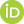## Publication Details

 Category Text Publication Reference Category Journals DOI 10.1021/ci800253u Title (Primary) External validation and prediction employing the predictive squared correlation coefficient - test set activity means vs training set activity mean Author Schüürmann, G.; Ebert, R.U.; Chen, J.; Wang, B.; Kühne, R.Journal Journal of Chemical Information and Modeling Year 2008 Department OEC Volume 48 Issue 11 Page From 2140 Page To 2145 Language englisch Abstract The external prediction capability of quantitative structure−activity relationship (QSAR) models is often quantified using the predictive squared correlation coefficient, q2. This index relates the predictive residual sum of squares, PRESS, to the activity sum of squares, SS, without postprocessing of the model output, the latter of which is automatically done when calculating the conventional squared correlation coefficient, r2. According to the current OECD guidelines, q2 for external validation should be calculated with SS referring to the training set activity mean. Our present findings including a mathematical proof demonstrate that this approach yields a systematic overestimation of the prediction capability that is triggered by the difference between the training and test set activity means. Example calculations with three regression models and data sets taken from literature show further that for external test sets, q2 based on the training set activity mean may become even larger than r2. As a consequence, we suggest to always use the test set activity mean when quantifying the external prediction capability through q2 and to revise the respective OECD guidance document accordingly. The discussion includes a comparison between r2 and q2 value ranges and the q2 statistics for cross-validation. Persistent UFZ Identifier https://www.ufz.de/index.php?en=20939&ufzPublicationIdentifier=1438 Schüürmann, G., Ebert, R.U., Chen, J., Wang, B., Kühne, R. (2008): External validation and prediction employing the predictive squared correlation coefficient - test set activity means vs training set activity mean J. Chem Inf. Model. 48 (11), 2140 - 2145